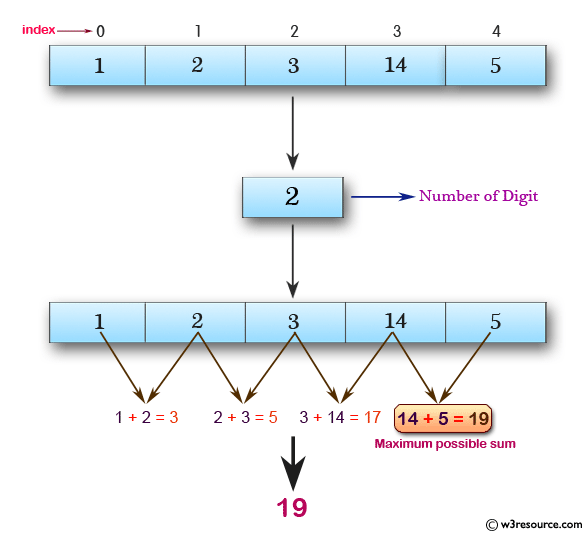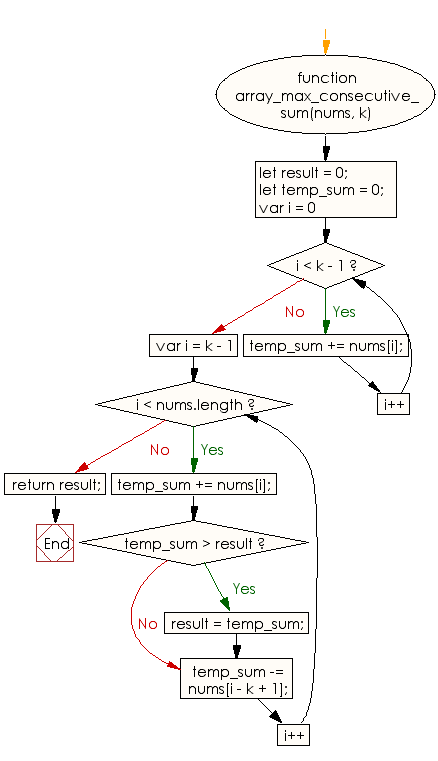# JavaScript: Find the maximum possible sum of some of its k consecutive numbers of a specified array of positive integers

## JavaScript Basic: Exercise-91 with Solution

Write a JavaScript program to find the maximum possible sum of some of its k consecutive numbers (numbers that follow each other in order.) of a given array of positive integers.

Pictorial Presentation:Sample Solution:

HTML Code:

``````<!DOCTYPE html>
<html>
<meta charset="utf-8">
<meta name="viewport" content="width=device-width">
<title>Find the maximum possible sum of some of its k consecutive numbers of a specified array of positive integers.</title>
<body>

</body>
</html>
```
```

JavaScript Code:

``````function array_max_consecutive_sum(nums, k) {
let result = 0;
let temp_sum = 0;
for (var i = 0; i < k - 1; i++) {
temp_sum += nums[i];
}
for (var i = k - 1; i < nums.length; i++) {
temp_sum += nums[i];
if (temp_sum > result) {
result = temp_sum;
}
temp_sum -= nums[i - k + 1];
}
return result;
}

console.log(array_max_consecutive_sum([1, 2, 3, 14, 5], 2))
console.log(array_max_consecutive_sum([2, 3, 5, 1, 6], 3))
console.log(array_max_consecutive_sum([9, 3, 5, 1, 7], 2))
```
```

Sample Output:

```19
12
12
```

Flowchart:ES6 Version:

``````function array_max_consecutive_sum(nums, k) {
let result = 0;
let temp_sum = 0;
for (var i = 0; i < k - 1; i++) {
temp_sum += nums[i];
}
for (var i = k - 1; i < nums.length; i++) {
temp_sum += nums[i];
if (temp_sum > result) {
result = temp_sum;
}
temp_sum -= nums[i - k + 1];
}
return result;
}

console.log(array_max_consecutive_sum([1, 2, 3, 14, 5], 2))
console.log(array_max_consecutive_sum([2, 3, 5, 1, 6], 3))
console.log(array_max_consecutive_sum([9, 3, 5, 1, 7], 2))
``````

Live Demo:

See the Pen javascript-basic-exercise-91 by w3resource (@w3resource) on CodePen.

What is the difficulty level of this exercise?

Test your Programming skills with w3resource's quiz.

﻿

## JavaScript: Tips of the Day

Returns the sum of the powers of all the numbers from start to end (both inclusive)

Example:

```const sumPower = (end, power = 2, start = 1) =>
Array(end + 1 - start)
.fill(0)
.map((x, i) => (i + start) ** power)
.reduce((a, b) => a + b, 0);
console.log(sumPower(10)); // 385
console.log(sumPower(10, 3)); // 3025
console.log(sumPower(10, 3, 5)); // 2925
```

Output:

```385
3025
2925
```